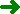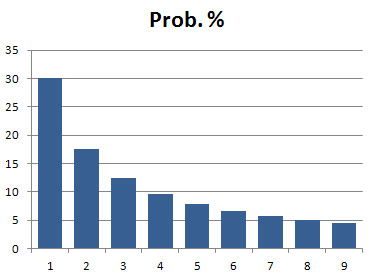Torsten Meyer HomepageFysik og matematikProbability of first significant digit

# Probability distribution of first significant digit

 Given a list of numbers from data extracted from a "real life source". What is the probability of the first significant digit to be '1', '2', ... ,'9'? The answer depends on the data chosen, but surprisingly the probability distribution is in many cases not even - as many would expect - but following a specific logartihmic distribution. In the first years of my Physics study (1965...), we had to use logarithmic tables with eight decimal places for our calculations, and I wondered at the fact that the first pages in that rather voluminous book were much more worn than the pages in the middle and the end of the book. I could not imagine that the reason for this could be that the users of these tables turned pages from the first page and on looking-up the requested number entrance. I did not follow this matter up, but later I became aware of that the American astronomer Simon Newcomb had noticed the same pecularity and proposed a probability distribution law for the first significant digits. For data containing a reasonable large number of numbers the distribution in many cases look like this:The probability of the first significant digit to be 1 is almost 1/3 (30,1%) and to be 1, 2 or 3 is more than 50% (60,2%)! The law tends to apply better if the data values covers many orders of magnitude (that is many different powers of 10). The half-life periods of decaying radioactive substances is a very good example of this. It is evident that not all kinds of data will fulfil this distribution law. A table of grown-up people's weights in kg, evidently will have quite a different distribution concerning the first significant figure. To explain this phenomena you need to do some assumptions. The most common explanation is based on the assumption that, given data extracted from some source from "real life", the distribution of the first significant digit must be independent of the unit system. For example, given a table of half-life periods of radioactive substances, it would be strange if the distribution changed strikingly by changing the unit of time from seconds to hours. The mathematical proof that this assumption leads to a specific distribution as graphed above is given in this document: FirstSignDig111212.pdf In practice, to get a stochastically significant result, the table of data must be large and cover a large range of orders of magnitude. (Actually for instance a table of half-life periods of all nuclear elements can even be presenting the data in many different time-units, from micro-seconds to thousands of millions of years (109 years), and the data will still fulfil the distribution law with very high accuracy.) See also: http://en.wikipedia.org/wiki/Benford's_law, which contain many further references. This site as downloaded 5-12-2011: BenfordsLawWikipedia111205.pdf

12-12-2011